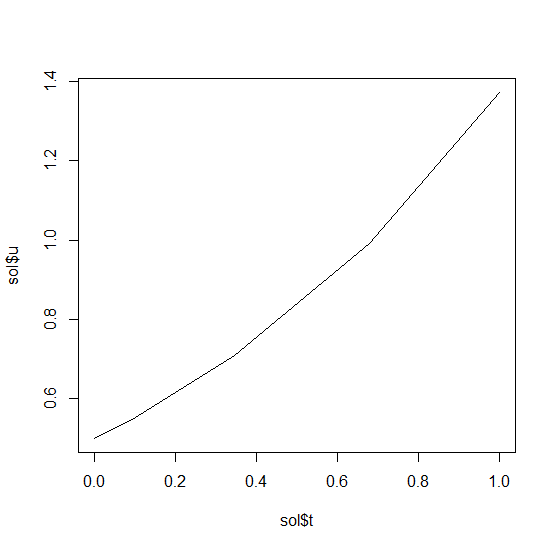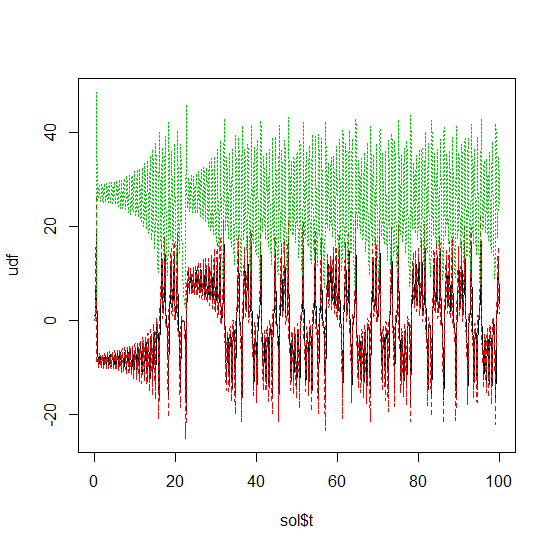Solving Ordinary Differential Equations (ODE) in R with diffeqr

1D Linear ODEs

Let’s solve the linear ODE u'=1.01u. First setup the package:

de <- diffeqr::diffeq_setup()

Define the derivative function f(u,p,t).

f <- function(u,p,t) {
return(1.01*u)
}

Then we give it an initial condition and a time span to solve over:

u0 <- 1/2
tspan <- c(0., 1.)

With those pieces we define the ODEProblem and solve the ODE:

prob = de\$ODEProblem(f, u0, tspan)
sol = de\$solve(prob)

This gives back a solution object for which sol\$t are the time points and sol\$u are the values. We can treat the solution as a continuous object in time via

and a high order interpolation will compute the value at t=0.2. We can check the solution by plotting it:

plot(sol\$t,sol\$u,"l")linear_ode

Systems of ODEs

Now let’s solve the Lorenz equations. In this case, our initial condition is a vector and our derivative functions takes in the vector to return a vector (note: arbitrary dimensional arrays are allowed). We would define this as:

f <- function(u,p,t) {
du1 = p*(u-u)
du2 = u*(p-u) - u
du3 = u*u - p*u
return(c(du1,du2,du3))
}

Here we utilized the parameter array p. Thus we use diffeqr::ode.solve like before, but also pass in parameters this time:

u0 <- c(1.0,0.0,0.0)
tspan <- list(0.0,100.0)
p <- c(10.0,28.0,8/3)
prob <- de\$ODEProblem(f, u0, tspan, p)
sol <- de\$solve(prob)

The returned solution is like before except now sol\$u is an array of arrays, where sol\$u[i] is the full system at time sol\$t[i]. It can be convenient to turn this into an R matrix through sapply:

mat <- sapply(sol\$u,identity)

This has each row as a time series. t(mat) makes each column a time series. It is sometimes convenient to turn the output into a data.frame which is done via:

udf <- as.data.frame(t(mat))

Now we can use matplot to plot the timeseries together:

matplot(sol\$t,udf,"l",col=1:3)timeseries

Now we can use the Plotly package to draw a phase plot:

plotly::plot_ly(udf, x = ~V1, y = ~V2, z = ~V3, type = 'scatter3d', mode = 'lines')